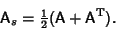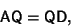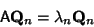## Symmetric Matrix

A symmetric matrix is a Square Matrix which satisfieswheredenotes the Transpose, so. This also implies(1)

where I is the Identity Matrix. Written explicitly,(2)

The symmetric part of any Matrix may be obtained from(3)

A Matrix A is symmetric if it can be expressed in the form(4)

whereis an Orthogonal Matrix andis a Diagonal Matrix. This is equivalent to the Matrix equation(5)

which is equivalent to(6)

for all, where. Therefore, the diagonal elements ofare the Eigenvalues of, and the columns ofare the corresponding Eigenvectors.

Nash, J. C. Real Symmetric Matrices.'' Ch. 10 in Compact Numerical Methods for Computers: Linear Algebra and Function Minimisation, 2nd ed. Bristol, England: Adam Hilger, pp. 119-134, 1990.